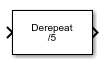# Derepeat

Reduce sampling rate by averaging consecutive samples

• Library:
• Communications Toolbox / Sequence Operations

•## Description

The Derepeat block resamples the discrete input at a rate 1/N times the input sample rate by averaging N consecutive samples. N represents the ```Derepeat factor, N``` parameter.

## Ports

### Input

expand all

Input signal, specified as a scalar, vector, or matrix.

Data Types: `double`
Complex Number Support: Yes

### Output

expand all

Output signal, returned as a scalar or column vector.

Data Types: `double`
Complex Number Support: Yes

For more information on the processing rates, see Single-Rate Processing, and Multirate Processing.

## Parameters

expand all

Derepeat factor, specified as an integer. The derepeat factor is the number of consecutive input samples to average to produce each output sample.

Data Types: `double`

Input processing control, specified as one of these options:

• `Columns as channels (frame based)` — The block treats each column of the input as a separate channel.

• `Elements as channels (sample based)` — The block treats each element of the input as a separate channel.

Block processing rate, specified as one of these options:

• `Allow multirate processing` — The block downsamples the signal such that the output sample rate is `Derepeat factor, N` times slower than the input sample rate. For more information, see Multirate Processing.

• `Enforce single-rate processing` — The block maintains the input sample rate by decreasing the output frame size by a factor equal to the ```Derepeat factor, N``` parameter value. Also, in single-rate processing mode you can use this block in a triggered subsystem. For more information, see Single-Rate Processing

Initial condition, specified as a scalar, vector, or matrix. This parameter specifies values that are output when it is too early for the input data to show up in the output. If the dimensions of the Initial condition parameter match the output dimensions, then the parameter represents the initial output value. If Initial condition is a scalar, then it represents the initial value of each element in the output. The block does not support empty matrices for initial conditions.

Data Types: `double`

## Block Characteristics

 Data Types `double` | `single` Multidimensional Signals `no` Variable-Size Signals `no`

expand all